Question

# 1. Derive the transfer function for the circuit shown below. Plot FH(s) versus frequency in Hertz,...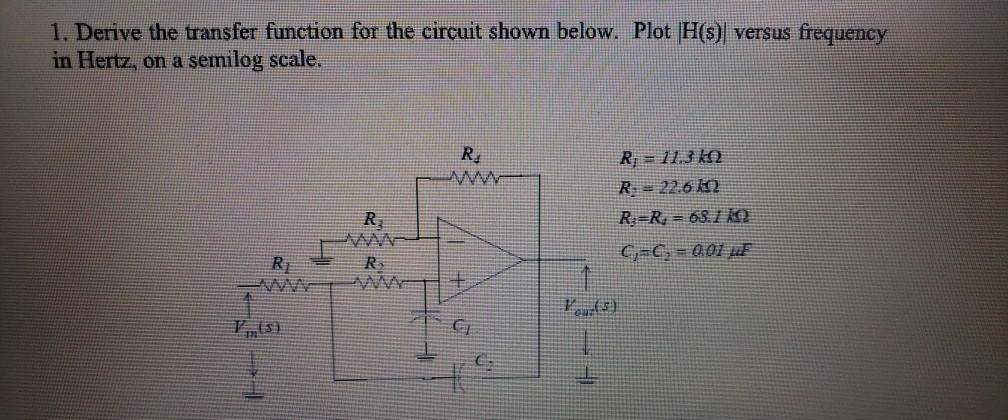1. Derive the transfer function for the circuit shown below. Plot FH(s) versus frequency in Hertz, on a semilog scale. Re R 113 k R 22.6 12 R R 63.12 Rj F)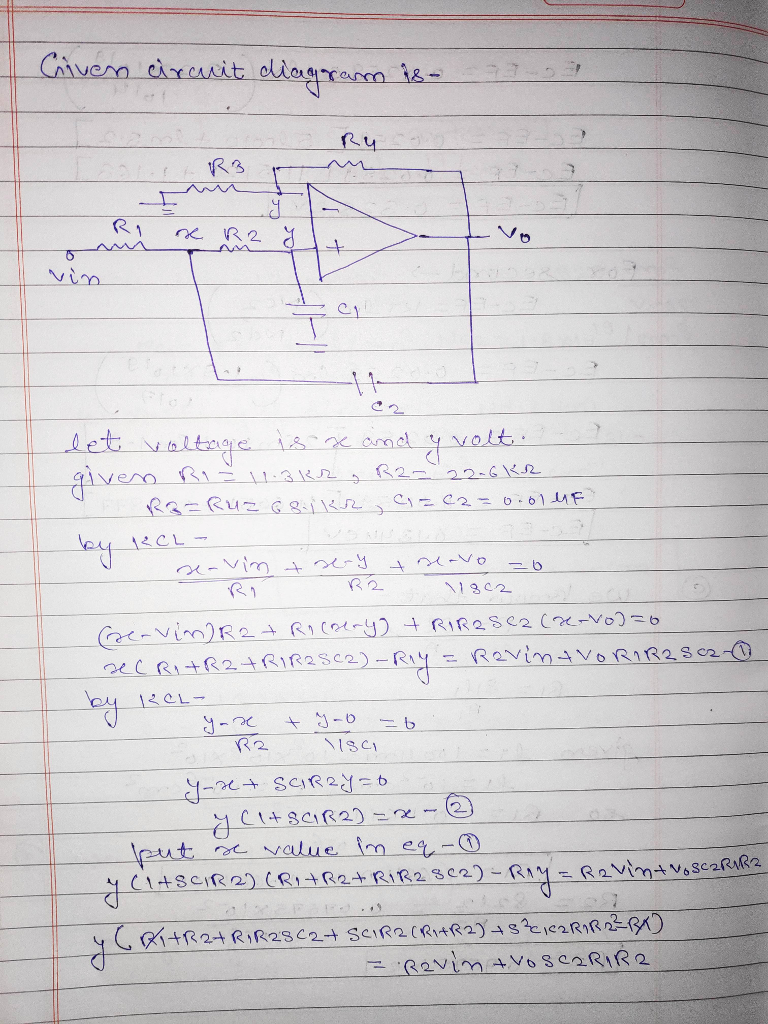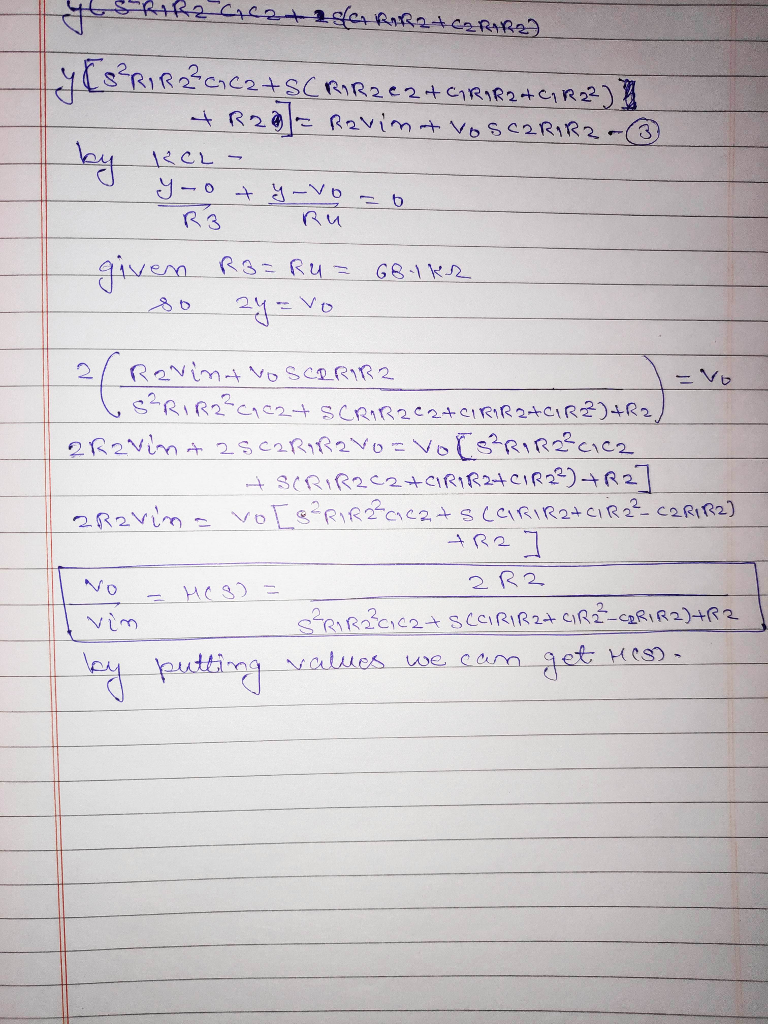#### Earn Coins

Coins can be redeemed for fabulous gifts.

Similar Homework Help Questions
• ### QUESTION #2 PLEASE 1. Derive the transfer function for the circuit shown below. Plot H(s) versus...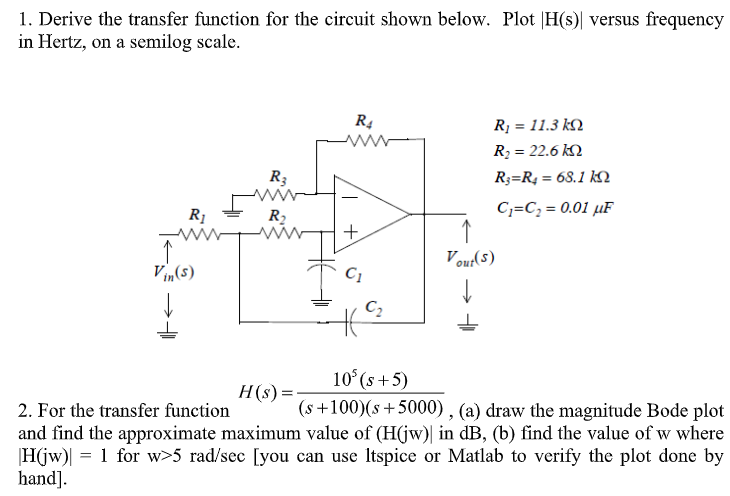QUESTION #2 PLEASE 1. Derive the transfer function for the circuit shown below. Plot H(s) versus frequency in Hertz, on a semilog scale. Ri 11.3 k Ri 22.6 k R R = 68.1 kN R3 C C 0.01 uF R2 Vout(s) Vin(s) C2 10 (s+5) H(s) = (s+100)(s5000) , (a) draw the magnitude Bode plot 2. For the transfer function and find the approximate maximum value of (H(jw) in dB, (b) find the value of w where 1 for w>5...

• ### Determine the complex transfer function T(s) = V/V; for the circuit shown below. Specify it as...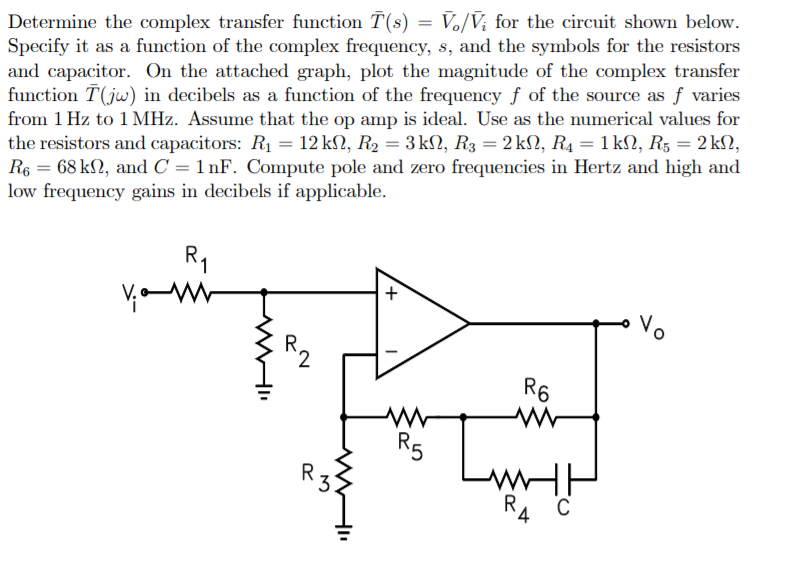Determine the complex transfer function T(s) = V/V; for the circuit shown below. Specify it as a function of the complex frequency, s, and the symbols for the resistors and capacitor. On the attached graph, plot the magnitude of the complex transfer function T(jw) in decibels as a function of the frequency f of the source as f varies from 1 Hz to 1 MHz. Assume that the op amp is ideal. Use as the numerical values for the resistors...

• ### For the circuit shown below, a) Determine the transfer function vo/vi b) Plot lVolvil versus ω...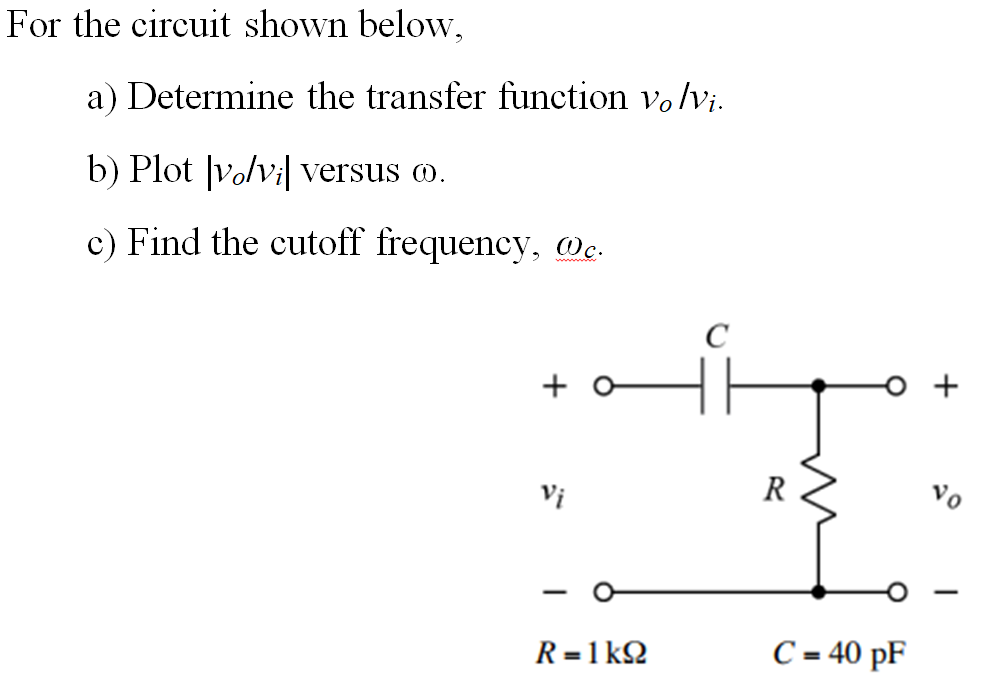For the circuit shown below, a) Determine the transfer function vo/vi b) Plot lVolvil versus ω c) Find the cutoff frequency, n. Vi C=40pF

• ### Q11 (20pts.): For the circuit shown below Derive the transfer function, H(s) assuming the OPAMP's transfer...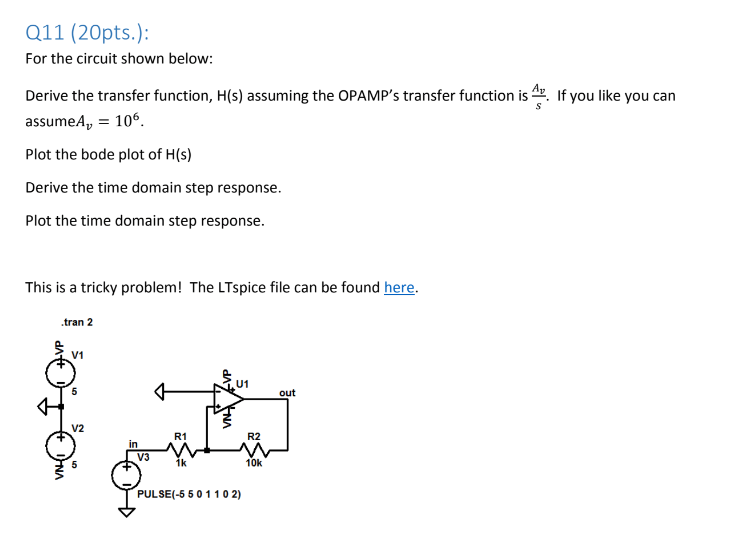Q11 (20pts.): For the circuit shown below Derive the transfer function, H(s) assuming the OPAMP's transfer function is. If you like you can assumeA 106 Plot the bode plot of H(s) Derive the time domain step response. Plot the time domain step response This is a tricky problem! The LTspice file can be found here. .tran 2 V1 U1 out V2 R1 R2 in V3 1k 10k PULSE(-5 50 1102)

• ### 1.6 For the circuit shown, derive the resonance frequency and plot the resonance frequency behavior as...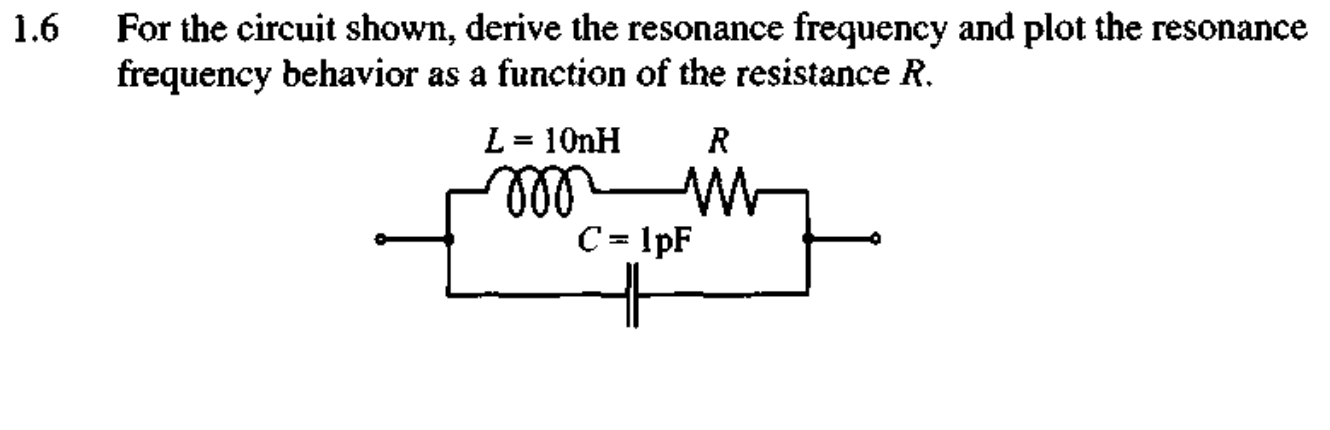1.6 For the circuit shown, derive the resonance frequency and plot the resonance frequency behavior as a function of the resistance R. L = 10nH R C = 1pF

• ### the circuit shown, 1. Find the transfer function H(jw) 2. If R R2 12 and L1mH, plot the frequency response (both the ga...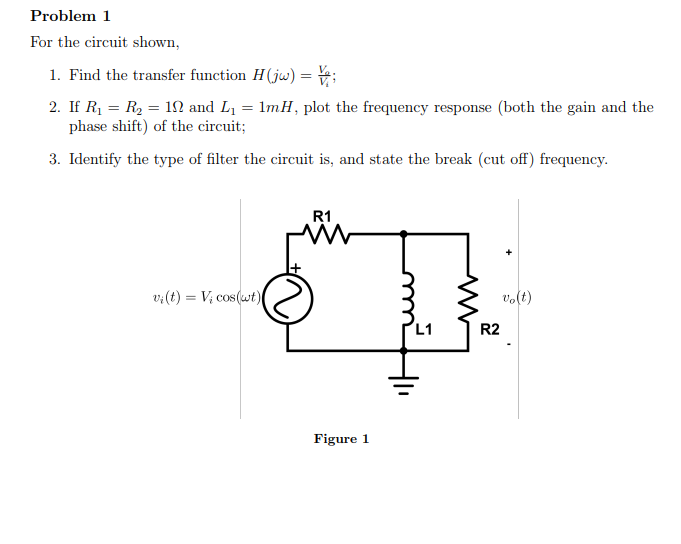the circuit shown, 1. Find the transfer function H(jw) 2. If R R2 12 and L1mH, plot the frequency response (both the gain and the phase shift) of the circuit; 3. Identify the type of filter the circuit is, and state the break (cut off) frequency. R1 v(t)Vcos(ut) L1 R2 Figure 1 the circuit shown, 1. Find the transfer function H(jw) 2. If R R2 12 and L1mH, plot the frequency response (both the gain and the phase shift) of...

• ### Problem 1: (Time Response) Derive the transfer function (s) of the electrical circuit shown below. Then...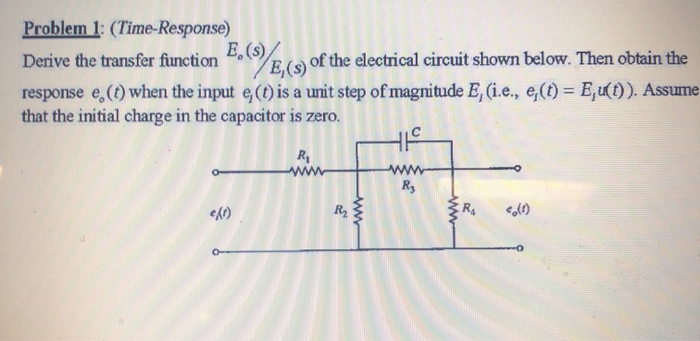Problem 1: (Time Response) Derive the transfer function (s) of the electrical circuit shown below. Then obtain the response e (0) when the input (1) is a unit step of magnitude E, (i.e., e(t) = E, (t)). Assume that the initial charge in the capacitor is zero. e/o)

• ### Pre-Laboratory Task 4: Derive an expression for the magnitude of the transfer function, H(Go)Vout(jo)/Wn(j, and the phase of the transfer function LH (ja) for the LCR circuit in Figure 4. Plot H(ja)l...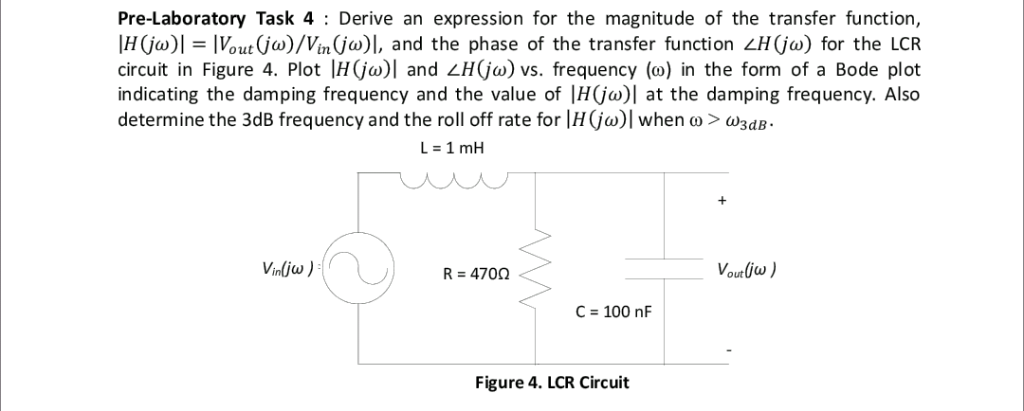Pre-Laboratory Task 4: Derive an expression for the magnitude of the transfer function, H(Go)Vout(jo)/Wn(j, and the phase of the transfer function LH (ja) for the LCR circuit in Figure 4. Plot H(ja)l and H(jo) vs. frequency (o) in the form of a Bode plot indicating the damping frequency and the value of |H(jo)| at the damping frequency. Also determine the 3dB frequency and the roll off rate for Ir(ja)1 when ω > ω3dB. Vounlius R 470Ω C 100 nF Figure...

• ### Find the transfer function H(s) for the circuit below. Make s=jw and plot the Bode plot...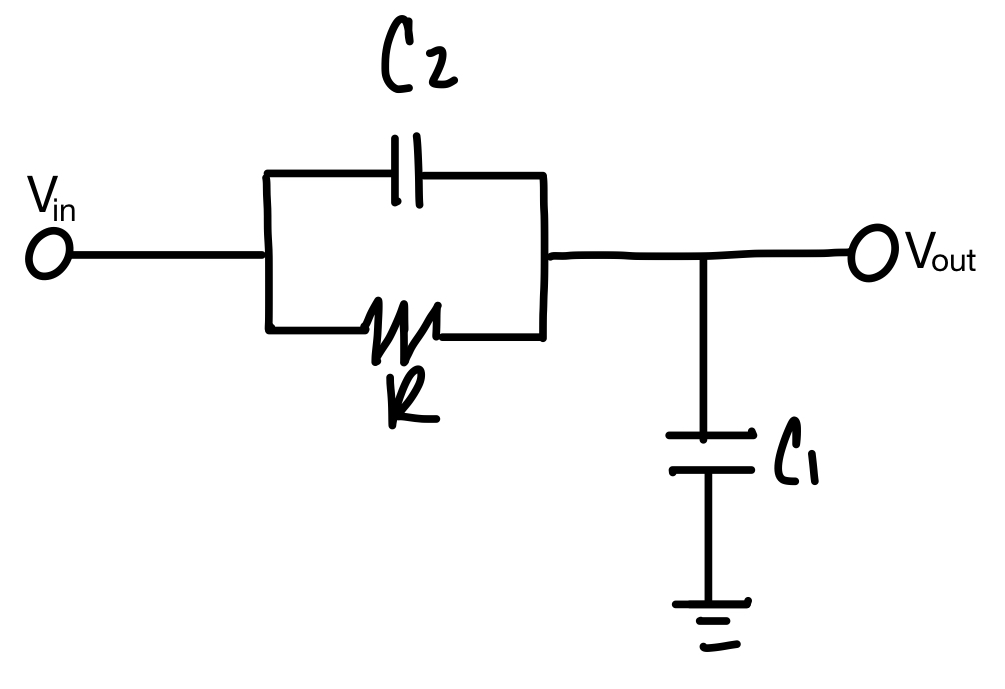Find the transfer function H(s) for the circuit below. Make s=jw and plot the Bode plot (magnitude and phase response) for the transfer function. C = out 페

• ### L Consider the circuit shown below by Using the traar fanction found in pat (ak derive an cxpress...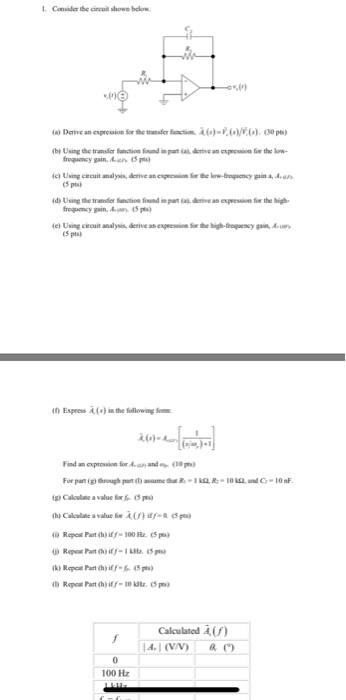L Consider the circuit shown below by Using the traar fanction found in pat (ak derive an cxpression for the ow c) Using circuit analysis, derive an epssio o te lorw-froquency gain a, Aun (5 pts (d) Using the transfer fatile fundi.~ส6aa.deme an epression fr the hi froguency gain, (5p) e) Using cecuit analysis, derive an expssion o te high-frogency ga,A (5 pts) Expeess A (s) in the following o Find an exprossion for Aan ande (30pes For parig,through h@aunetha...